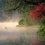# geometry question

Might be simple for some of you but pls type solution as I couldnt solve

1) In $\triangle ABC,\ AD,BE,CF$ are concurrent cevians and $AD$ is altitude.

Then prove that $AD$ bisects $\angle FDE$Note by Megh Parikh
6 years, 6 months ago

This discussion board is a place to discuss our Daily Challenges and the math and science related to those challenges. Explanations are more than just a solution — they should explain the steps and thinking strategies that you used to obtain the solution. Comments should further the discussion of math and science.

When posting on Brilliant:

• Use the emojis to react to an explanation, whether you're congratulating a job well done , or just really confused .
• Ask specific questions about the challenge or the steps in somebody's explanation. Well-posed questions can add a lot to the discussion, but posting "I don't understand!" doesn't help anyone.
• Try to contribute something new to the discussion, whether it is an extension, generalization or other idea related to the challenge.

MarkdownAppears as
*italics* or _italics_ italics
**bold** or __bold__ bold
- bulleted- list
• bulleted
• list
1. numbered2. list
1. numbered
2. list
Note: you must add a full line of space before and after lists for them to show up correctly
paragraph 1paragraph 2

paragraph 1

paragraph 2

[example link](https://brilliant.org)example link
> This is a quote
This is a quote
    # I indented these lines
# 4 spaces, and now they show
# up as a code block.

print "hello world"
# I indented these lines
# 4 spaces, and now they show
# up as a code block.

print "hello world"
MathAppears as
Remember to wrap math in $$ ... $$ or $ ... $ to ensure proper formatting.
2 \times 3 $2 \times 3$
2^{34} $2^{34}$
a_{i-1} $a_{i-1}$
\frac{2}{3} $\frac{2}{3}$
\sqrt{2} $\sqrt{2}$
\sum_{i=1}^3 $\sum_{i=1}^3$
\sin \theta $\sin \theta$
\boxed{123} $\boxed{123}$

Sort by:

Hint for (1): Draw the line $\ell$ parallel to $BC$ through $A$. Suposse $DE$ and $DF$ meet $\ell$ at $P$ and $Q$.

- 6 years, 6 months ago

thanks

- 6 years, 6 months ago

Solution to #1 We drop $FX \perp BC$ and $EY \perp BC$. We need to show that

$\angle FDA = \angle ADE$

$\iff$ $\angle FDX = \angle EDY$

$\iff$ $\tan \angle FDX = \tan \angle EDY$

$\iff$ $\boxed{\dfrac{FX}{DX} = \dfrac{ EY}{DY}}$ ... $(i)$ . Thus if we can prove that (i) is true, we can claim that $AD$ bisects $\angle FDE$

Now, Ceva's theorem in $\triangle ABC$ we have

$\dfrac{BD}{DC} \times \dfrac{CE}{EA} \times \dfrac{AF}{FB} = 1$.... $(ii)$

Now, $\triangle FBX \sim \triangle ABD$ and $\triangle ECY \sim \triangle ACD$ and so we have after some computation, $FX = \dfrac{BX}{BD} \times AD$ and $EY = \dfrac{CY}{CD} \times AD$.

Therefore, $\dfrac{FX}{EY} = \dfrac{BX \times CD}{DB \times CY}$... $(iii)$ Coming back to $ii)$ and putting $\dfrac{CE}{EA} = \dfrac{CY}{YD}$ and $\dfrac{AF}{FB} = \dfrac{DX}{BX}$ we have

$\dfrac{BD}{DC} \times \dfrac{CE}{EA} \times \dfrac{AF}{FB} = 1$

$\implies$ $\dfrac{BD}{DC} \times \dfrac{CY}{YD} \times \dfrac{DX}{XB} = 1$

$\implies$ $\dfrac{DX}{DY} = \dfrac{BX \times DC}{CY \times BD}$ ....$(iv)$. Equating $(iii)$ and $(iv)$, we have

$\dfrac{DX}{DY} = \dfrac{FX}{EY}$ and thus we have $\boxed{\dfrac{FX}{DX} = \dfrac{ EY}{DY}}$. Thus we proved $(i)$ and hence we are done! :)

- 6 years, 6 months ago

i solved the question no. 2.. please see the solution and tell me whether it is correct or not :

in triangle ABC , FM = 2 and MC = 4 thus FM/MC = 1/2 also BE which is the median passes through M. Thus we conclude that M is the centroid of triangle ABC. thus as AD is perpendicular to BC and CF is angle bisector of triangle ABC, therefore triangle ABC is equilateral. thus in triangle ACF ,by Pythagoras theorem we have AB^2 = 36 + AB^2/4 or AB = 12/3^1/2 THUS PERIMETER OF TRIANGLE ABC = 12 TIMES ROOT OF 3

- 6 years, 6 months ago

correct!

- 6 years, 6 months ago

very clever. Better than mine solution.

- 6 years, 5 months ago

One more hint prove triangle QAP AND PAD similar By using cevians theorem and parallel lines

- 6 years, 6 months ago

Well, problem 2 has many solutions i think as i got 2 solutions. The first one requires to use the formula of the length of the angle bisector repeatedly. This will finally fetch you that $\triangle ABC$ is equilateral. That is the aim of the problem actually. Prove that $\triangle ABC$ is equilateral. If u cannot do just post a comment, Il type out the detailed solution for you.

- 6 years, 6 months ago

My new problem I created due to my misread of Q2

- 6 years, 6 months ago

by the way megha did you qualified for INMO?

- 6 years, 6 months ago

yes and not megha, "Megh"

- 6 years, 6 months ago

Problem 1 is really simple if u know the concept of harmonic bundles. The official solution is completely unmotivated

- 4 years, 4 months ago